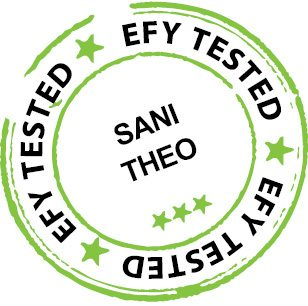Breaking News

# Image Processing Using MATLAB | Source Code IncludedIn this article, the author describes basic image processing using MATLAB software.

MATLAB is a high-performance language for technical computing with powerful commands and syntax. It is used for many purposes like Maths and computation, data analysis, algorithm development, modelling stimulation and prototyping. Edge detection, noise and image histogram modelling are some important and basic topics in image processing.

## Image processing using MATLAB

### Edge detection

An image is nothing but mapping of intensity of the light reflecting from a scene captured from a camera, and edges are the discontinuity of the scene intensity function. We can detect these edges using MATLAB commands. There are many methods for edge detection such as Robert’s operator, Prewitt operator, Sobel operator, Canny edge detector and so on. Fig. 1 shows edge detection using these operators on cameraman.tif standard image available in MATLAB.

Noise in any system is unwanted. In image processing, noise in a digital image arises during image acquisition and also during transmission. Different types of noise include speckle, Gaussian, salt-and-pepper and more. The fun part is, we can use these types of noise as special effects in an image using MATLAB.

Fig. 2 shows the results of different types of noise added to an image. In this image, RGB-to-gray conversion is done first and then different types of noise are added in the image through the program. All operations are included in MATLAB program.

### Histogram modelling

A histogram of an image provides a vast description about an image. It represents the occurrence of various gray levels relative to the frequencies. In this program, we plot the histogram of the original image and of the histogram-equalised image.

Running the program is straightforward. There are three .m files, one each for edge detection, noise effects and histogram. Two image files (.jpeg) are also included along with these .m files in the same folder. Launch MATLAB R2013a from your desktop and open an .m file from C:\Users\SONY\Desktop folder to run the program.

Image processing is a diverse and the most useful field of science, and this article gives an overview of image processing using MATLAB. There are many more topics that are useful and can be applied using MATLAB or OpenCV library such as erosion, dilation, thresholding, smoothing, degradation and restoration, segmentation part like point processing, line processing and edge detection (covered here) of images.